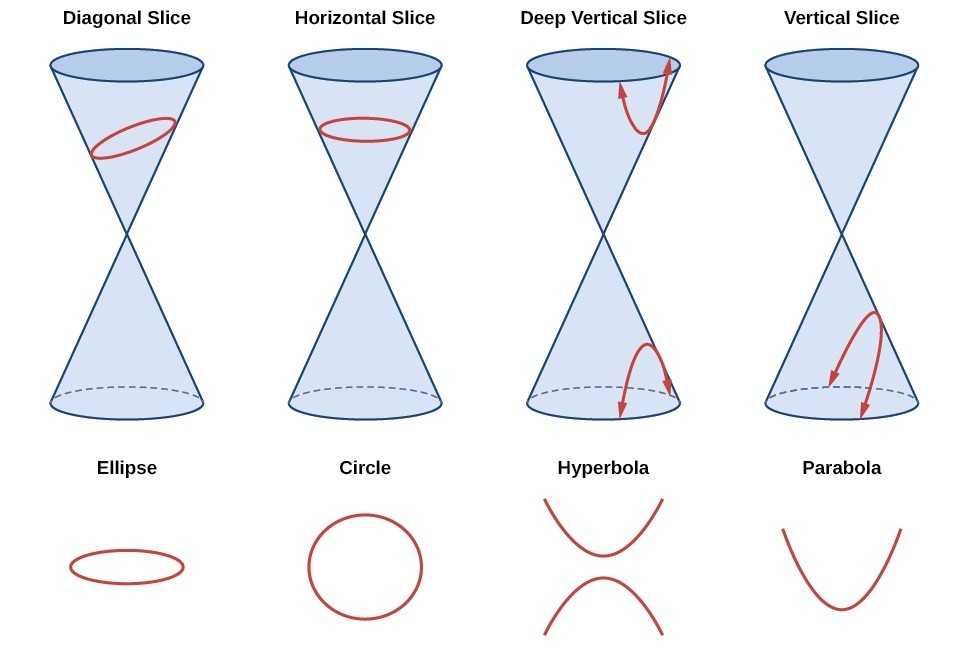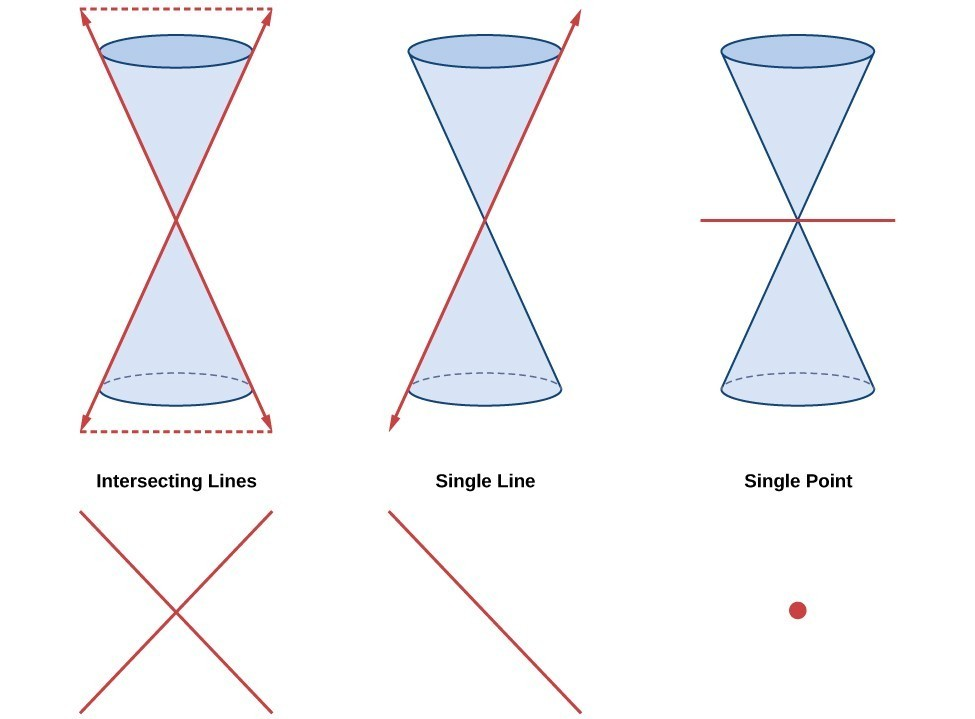## Introduction to Rotation of Axes

### Learning Objectives

By the end of this section, you will be able to:

• Identify nondegenerate conic sections given their general form equations.
• Use rotation of axes formulas.
• Write equations of rotated conics in standard form.
• Identify conics without rotating axes.

As we have seen, conic sections are formed when a plane intersects two right circular cones aligned tip to tip and extending infinitely far in opposite directions, which we also call a cone. The way in which we slice the cone will determine the type of conic section formed at the intersection. A circle is formed by slicing a cone with a plane perpendicular to the axis of symmetry of the cone. An ellipse is formed by slicing a single cone with a slanted plane not perpendicular to the axis of symmetry. A parabola is formed by slicing the plane through the top or bottom of the double-cone, whereas a hyperbola is formed when the plane slices both the top and bottom of the cone.Figure 1. The nondegenerate conic sections

Ellipses, circles, hyperbolas, and parabolas are sometimes called the nondegenerate conic sections, in contrast to the degenerate conic sections, which are shown in Figure 2. A degenerate conic results when a plane intersects the double cone and passes through the apex. Depending on the angle of the plane, three types of degenerate conic sections are possible: a point, a line, or two intersecting lines.Figure 2. Degenerate conic sections